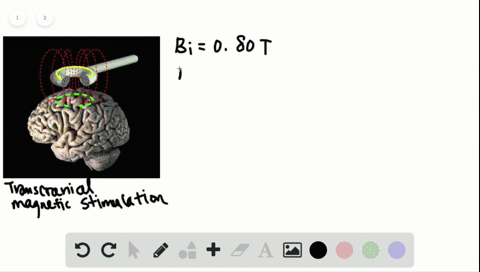🎉 The Study-to-Win Winning Ticket number has been announced! Go to your Tickets dashboard to see if you won! 🎉View Winning Ticket### Stimulating the brain In transcranial magnetic st…

04:57Indian Institute of Technology Kharagpur
Problem 19

# sA magnetic field passing through two identical coils decreases from a magnitude of $B_{\text { ex }}$ to zero in the time interval $\Delta t$ . The first coil has twice the number of turns as the second. (a) Compare the emfs induced in the coils.(b) How can you change the experiment so that the emfs produced in them are the same?

## Discussion

You must be signed in to discuss.

## Video Transcript

e m f induced in a coil is given by e equals negative D C d t equals negative de de de off Number of targets Magnetic field area off the coil and go sign Terra is the angle Bedouin be on a So with respect to time, the coil number does not change. The area does not change. The orientation of the coil with respect to the magnetic field does not change. So this comes out to be in a go scientific data times negative d b t t. So if we have to compare the Yemen in used on quite a one versus Karl to it would be you won over E Do is equal to number of times in Korean Juan by a number of turns inquired too Area off. Both of them are same on initially there oriented identical t. So call scientist A for the first card is equal Giokos antenna for the second girl on Ben but died by negative TB t t for the first girl on negative db DT for the second car which will come out to be in one over and to because everything else is identical now it is given that it is given that the first card has got twice the number of times. So this is this value is to so answered off part A ese e m f induced inquired One equals twice the sheriff and used to inquire too. Now let us all the second part now, Part B. They're saying, how can you have you want equals be to one thing that I should mention over here, which is not important to this problem. Is changing magnetic fields divided by changing time equals Change in time is giving us delta t. So we don't have to worry about that. Whenever there is a change involved, it is always final minus initial final value off magnetic with zero initial valleys be x which comes out to be negative be e x over delta t We will not need this. We will not need this in this problem. But I just want to ah, give this sport to you all. Okay, Now how can we have you want because he to way do not We will not be able to change anything else other than the co sign terror over here. So I am going to write What will happen when you want. It was 82. We know that. We know that for in order to have you wanna equals e Do we have to have in the one times a time school sign Kidder one times the negative off dp DT which will come out to be? I don't want to write the ability anymore. However I can. But I am just going to write B e X over del dirty equals and to a co sign Ted are too. Be Yes, over. Tell the D. If we cancel all off the common terms, then we're left out with it in the one co sign. Ted Dewan equals. Remember, over here, we use any trick was to end two equals half and one. So you're going to use entry quiz half and one times co sign too. Oh, we find co sign that are too. It was too well saying Ted uh, one. So this is how we can change The orientation of the card is such that the oil that has got more number of turn has got half the footprint. There is one solution to this problem. Uh, if we dick ST R two equals nine territory equals zero degree Andi theater. One equals 60. Then co sign Tedder too. Because one Andi co scientist a one it was half. So this Ah, special guests How you can ah, orient the corn one such that it still gets 60 degree with respect to the magnetic field. So if we have the magnetic field coming in this direction, we're going to put the forced coil at 60 degree angle and we're going to put the second cars hit on. And second card has got twice that turnover here and the first girl has gone. I'm sorry, E. So they just redo the drawing. Are you Strack off? What is what If we have magnetic fields flowing in this direction, then the first card, which has got twice the number of stars, has to be positioned like this there to dance. You can see in this going and the first girl and the second guy has got only one turn half the darn and it is putting head on. So this is how you can have both the cars having same E m f induced on them